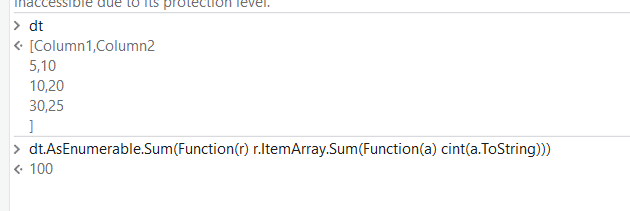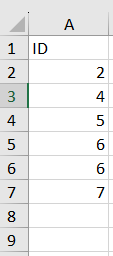# Sum of Datatable

Dear UiPath team,

I would like to sum my datatable values. I learned how to Concat the values, but is there a way to sum?

Sum of

1. Rows - (From row in dtSum.AsEnumerable Let val=(From item in row.ItemArray Select Cint(item)).ToList.Sum Select val).ToArray

2. Columns - (From col in dtSum.Columns Let val=(From row in dtSum Select Cint(row(col.ToString))).ToList.sum Select val).ToArray
Sum_of_Rows_Columns.xaml (6.7 KB)

Hi @uiStijn

dt.AsEnumerable.Sum(Function(r) r.ItemArray.Sum(Function(a) cint(a.ToString)))Hope this helps

Hi @uiStijn

Try this

TotalSum= dt.AsEnumerable().Sum(Function(row) Convert.ToDouble(row(“Amount”)))

I/P:for the learnings: [HowTo] - Exploring the LINQ Universe (VB.Net)

1 Like

This topic was automatically closed 3 days after the last reply. New replies are no longer allowed.